Next: Truncated Lattices Up: Order vs. Algebraic Topology Previous: Topological Considerations

## Cutsets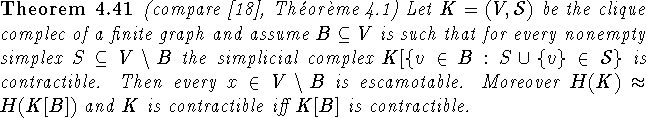Proof: This is an induction on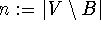. For n=0 there is nothing to prove. So now let n>0 and suppose the result holds for all k<n. Let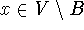and let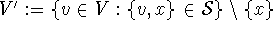,and let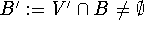. Then K' and B' satisfy the hypothesis of the theorem: In fact if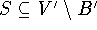is a simplex in K', then by the clique condition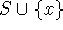is a simplex in K and again via the clique condition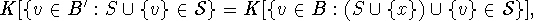where the latter simplicial complex is contractible. Moreover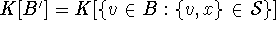is contractible by assumption. Now since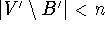by induction hypothesis K' is contractible and thus x is escamotable.
To prove the ``moreover" part we need to prove that K is ``dismantlable via escamotable points" to K[B]. To see this it is good enough to show that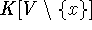and B satisfy the assumption of the theorem. Let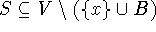be a simplex. Then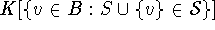is contractible by assumption and we are done. \

Clearly a set B as above must intersect every maximal simplex, which is exactly the notion of a cutset.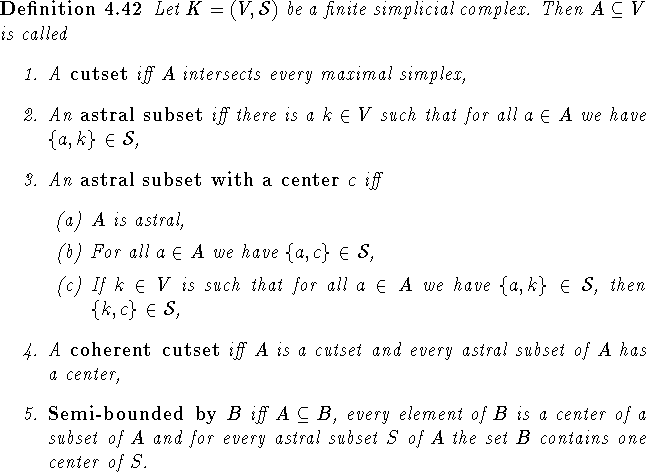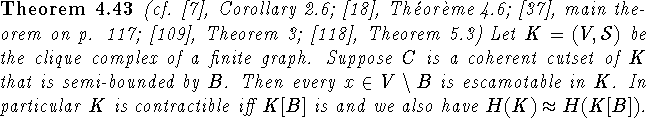Proof: We will prove that the simplicial complex satisfies the assumption of Theorem 4.41. Let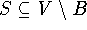be a simplex. Then the set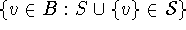has a center and is thus contractible. \

Bernd.S.W.Schroder